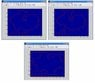# The discrete integral of a contour of an image

alitas
Hi everybody !

I have a question about discrete integrals with contours. I want to integrate
the points that makes the contour of an image. When the contour is only one
curve it is easy I get the function in every point of the contour and I multiply
by the distance between two consecutive points.

But my problems y when this curve changes of topology so the contour
can be several contours inside, outside the other curve. So I wonder if I can use the
same method.

Example 1: I calculate the corresponding functions
of the elements in the position of the contour multiplyed
by the width between two consecutive points.

000000000000
000011111100
000100000100
000010111100
000001000000

My problem Example 2: It is when my contour is not a curve
with starting and ending if not particionates and becomes
more curves. Someone knows how to calculate the discrete
integral over the points 1, and 2??.

0000000000000
0000111111000
0000102220000
0000102222220
0000100001120
0000111111120

The problem can be arbitrary I just show a case to explain my
problem

Thank you very much
Sincerely
Esmeralda Ruiz

My problem Example 2: It is when my contour is not a curve
with starting and ending if not particionates and becomes
more curves.

Do you mean that it "bifurcates" and becomes more curves?

It isn't possible to say what you should do unless we understand what you are trying to accomplish by doing the integration. What function are you integrating? What is the result of the integration supposed to mean?

alitas
Thanks for answering. I attached an example I want to calculate the integral of a contour (discrete points). The function could be the force that makes evolve this contour. The first example is the trivial I calculate the function in every points and I multiply it by the width between the two consecutive points so the results is ok (the last point with the first). The problem is when the curve changes of topology or as you said bifurcates in others caused by the force applying to it. Then I would still like to calculate the integral of that curve(s) to keep evolving until it converges. I do not know if there is any way to do it a numerical way to calculate discrete integrals over some points or numerical optimization methods. The ones I know they need a polinomial function not an image.

#### Attachments

•example 1.jpg
28.8 KB · Views: 429
The function could be the force that makes evolve this contour.

Does your problem represent an actual problem in physics (force = force in Newtons)?

Or is this a kind of metaphorical force - some function f(...) that you invented to measure how much a contour departs from a smooth shape like a circle, some function that merely plays a role in image processing?

alitas
It is the second thing the curve must evolve certain shape so the force can be for every point of the curve multiplied by a force. For example the force can be

c1 = average inside of the curve
c2 = average outside of Phi0
I1 = image
force=-(I1-c1).^2+(I1-c2).^2;

But the function I am using is working my problem is how to make the integral of
arbitrary points that can be in whatever point of the image. I also tried triangulization and getting the area of the triangles of every point. But it seems not work.

Thank you again

alitas
So for example if you have several x,y and z (function) that are not consecutive how to integrate
x,y,z=function
1,1,3
1,2,4
1,4,5

instead of having a polinomial function you have an arbitrary function.

Thanks again